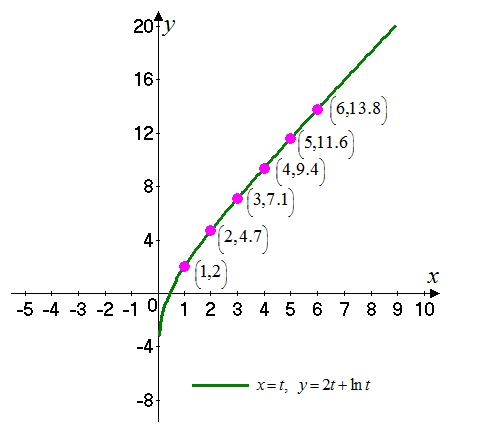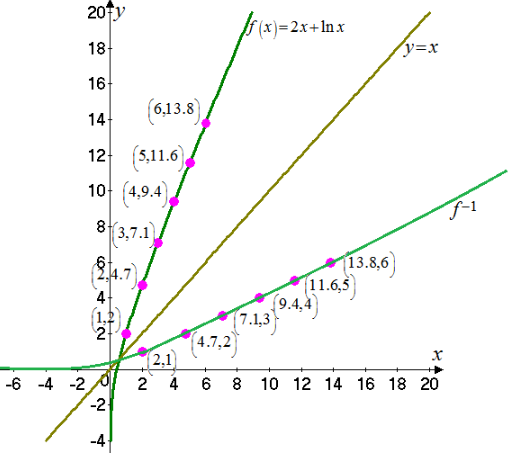# The graph for the given function and its parametric function### Single Variable Calculus: Concepts...

4th Edition
James Stewart
Publisher: Cengage Learning
ISBN: 9781337687805### Single Variable Calculus: Concepts...

4th Edition
James Stewart
Publisher: Cengage Learning
ISBN: 9781337687805

#### Solutions

Chapter 1, Problem 33RE
To determine

## To find: The graph for the given function and its parametric function

Expert Solution

### Explanation of Solution

Given:

The given parametric equation is f(x)=2x+lnx .

Calculation:

Consider the parameter is t=x .

Then the equation is,

x=ty=2t+lnt

The graph for the curve by the above parametric equations is shown in Figure 1Figure 1

The inverse of the graph f1 is the reflection of the graph f1(b) . As the value of f(a) equals b only when the points f1(b)=a when the points (a,b) on the graph of f and the points (b,a) are on the graph f1 .

Then the required function is,

f(x)=2x+lnx

The graph for the above function and the inverse function is shown in Figure 2Figure 2

### Have a homework question?

Subscribe to bartleby learn! Ask subject matter experts 30 homework questions each month. Plus, you’ll have access to millions of step-by-step textbook answers!